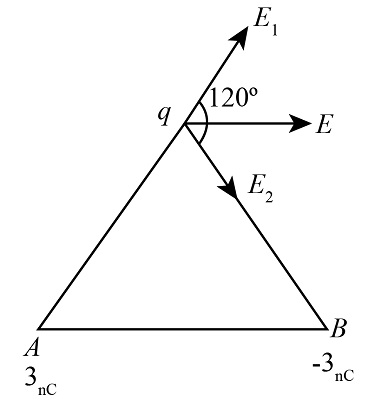# ABC is an equilateral triangle of side 0.1 m. Point charges of +3 nC and -3 nC are placed at the...

## Question:

ABC is an equilateral triangle of side 0.1 m. Point charges of +3 nC and -3 nC are placed at the corners A & B respectively. Calculate the resultant electric field at C.

## Electric field

The electric field is an electromagnetic concept involved in the movement of an electric charge. The electric field is a hypothetical concept that can only be experienced with the help of an experiment. The standardized unit to measure the electric field is Newton per inverse coulomb.

Given Data

• The length of the sides of equilateral triangle is {eq}l = 0.1\;{\rm{m}}. {/eq}
• The charge placed at point A is {eq}{q_A} = + 3\;{\rm{nC}}. {/eq}
• The charge placed at point B is {eq}{q_B} = - 3\;{\rm{nC}}. {/eq}The electric field due to point A is calculated as,

{eq}\begin{align*} {E_A} &= \dfrac{{kq}}{{{r^2}}}\\ &= \dfrac{{9 \times {{10}^9} \times 3 \times {{10}^{ - 9}}}}{{{{0.1}^2}}}\\ &= 2700\;{\rm{N/C}} \end{align*} {/eq}

The electric field due to point B is calculated as,

{eq}\begin{align*} {E_B} &= \dfrac{{kq}}{{{r^2}}}\\ &= - \dfrac{{9 \times {{10}^9} \times 3 \times {{10}^{ - 9}}}}{{{{0.1}^2}}}\\ &= - 2700\;{\rm{N/C}} \end{align*} {/eq}

Therefore the net electric at point C is calculated as,

{eq}{E_{net}} = \sqrt {E_A^2 + E_B^2 + 2{E_A}{E_B}\cos \theta } {/eq}

According to the diagram the {eq}\theta = 120^\circ . {/eq}

{eq}\begin{align*} {E_{net}} &= \sqrt {E_A^2 + E_B^2 + 2{E_A}{E_B}\cos \theta } \\ &= \sqrt {{{2700}^2} + {{\left( { - 2700} \right)}^2} + 2 \times 2700 \times \left( { - 2700} \right) \times \cos 120^\circ } \\ &= 4676.537\;{\rm{N/C}} \end{align*} {/eq}

Thus, the electric field at point C is 4676.537 N/C.